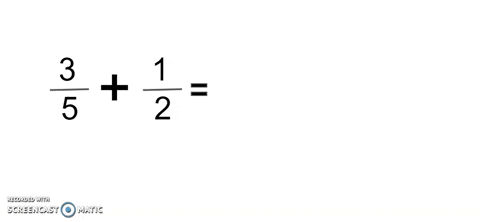# Division by ZERO is Undefined

I hope you all are aware of what is meant by division 😉 and you all know that dividing any given number by 0 is unfortunately impossible but some would think that any number divided by 0 is infinite (). Example: 10/0 = ∞, 5/0 = ∞

When it comes to computers, it doesn’t accept that any given number called X divided by 0 is infinite. So the problem is why the computer doesn’t accept this? Let’s try to figure it out.

We all are know how to perform with division and computer also uses their way to perform division. Now assume the computer wants to divide a given number. what it does is simply subtract the given number until it meets 0.

For example: Let’s try to with 20/4 & 8/2

To come up with an answer to the example 20/4, the computer must subtract 4 from 20, for 5 times to meet 0 also for the example 8/2, the computer must subtract 2 from 8, for 4 times to meet 0.

In the same way, when a computer performs multiplication, it does addition. For example, will take 4*5 & 2*4.

Now according to this, we are clear, If I take 20/4, the answer is 5. On the other way round, 5*4 is equal to 20 which means if dividend divided by divisor is equal to an answer called ‘y’. On the other hand, the answer called ‘y’ into divisor is equal to the dividend.

Let’s back to divide by ZERO

If we take a look at below,

According to the mathematical concept that we discussed, 10 divided by 0 is and 1 divided by 0 is also ∞. On the other way round, ∞ * 0 = 10 and * 0 is 1 so this simply means 1 = 10 which is not equal.

Therefore, any given number divided by 0 is not considered infinite().

Let’s have a look at why dividing by ZERO is undefined.

Now consider we want to divide 1 by 0, let’s divide 1 by positive decimal numbers and negative decimal numbers.

As we can see, when the number gets near zero and if it is positive then the result is a large value. When it comes to negative then the result takes a very small value. Let’s try to understand this by using a graph.

According to the above graph, we can see a & b ends going in different directions and c & d ends going in different directions as they never going to meet this is undefinable. Therefore we say any number divided by 0 is undefined. So, this is how we ended up “Division by ZERO is Undefined.”

--

--

--

## More from Roshni Silva

Associate Engineer at Virtusa

Love podcasts or audiobooks? Learn on the go with our new app.

## Properties of Discrete Random Variables## How I started liking Math and succeeded in Math courses at U of T## Index to Cosmology of Light Links## How to add fractions? I forgot!## Shamir’s Secret Sharing Step-By-Step## Why Probability is important in Data Science and Machine Learning?## John D’s Dixon’s Algorithm## Roshni Silva

Associate Engineer at Virtusa

## Best Electric Vehicles News Websites## CS373 Spring 2022: Presley Heikkila